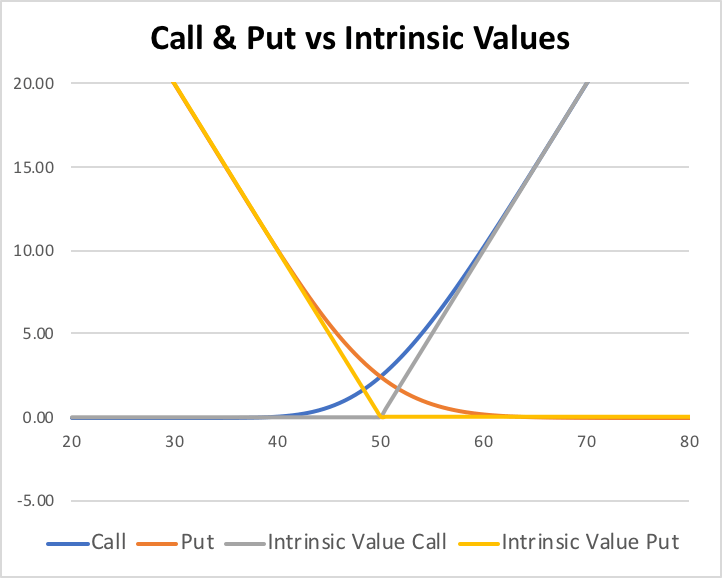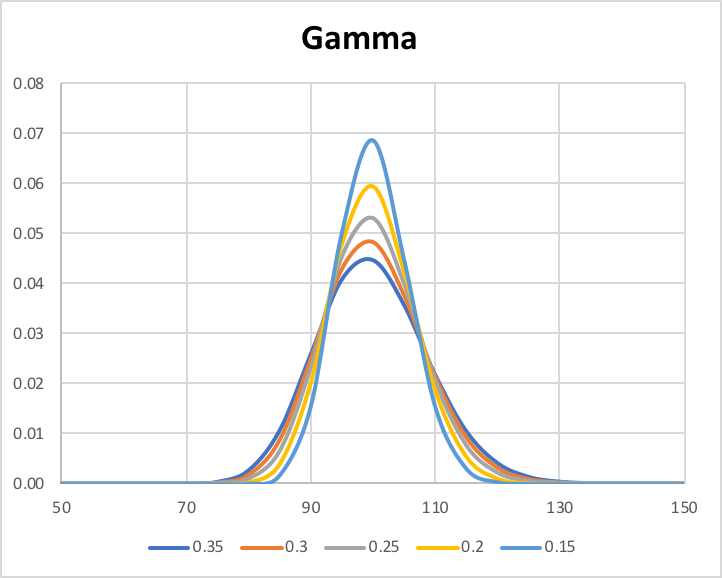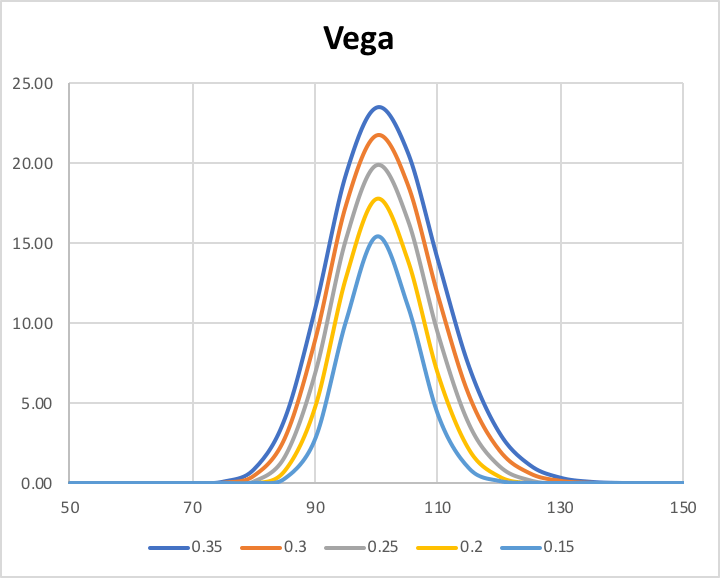The aim of this article is to walk the reader through the implementation of the Black-Scholes model for option pricing in VBA. Firstly, we’ll recap the theoretical framework. Secondly, we’ll provide the code to put the theory into practice and show some basic (but hopefully relevant) applications.

The Theory – Pricing

The classical Black-Scholes model for option pricing assumes that stock prices follow a Geometric Brownian Motion (GBM) with constant drift (μ) and constant volatility (σ). Analytically:

$\dfrac{dS(t)}{S(t)}=\mu dt + \sigma dW^P(t)$

where $W^P(t)$ is a Standard Brownian Motion with respect to the historical measure P.

It is possible to prove that for pricing purposes the equation becomes

$\dfrac{dS(t)}{S(t)}=(r-q)dt + \sigma dW^Q(t)$

where $W^Q(t)$ is a Standard Brownian Motion with respect to the risk-neutral measure Q and q is the continuous dividend yield. This SDE can be easily solved by applying Ito’s Lemma to the function ln(S(t)). The final result is

$dlnS(t) = (r-q-\dfrac{1}{2}\sigma^2)dt + \sigma dW^Q(t)$

which in integral notation is

$lnS(T)-lnS(t)=\int_t^T\left( r - q - \dfrac{1}{2}\sigma^2 \right) ds + \int_t^T \sigma dW^Q(s)$

that finally becomes

$S(T)=S(t)e^{\left( r - q - \dfrac{1}{2}\sigma^2 \right)\left(T-t\right) + \sigma\left(W^Q(T)-W^Q(t)\right)}$

Given the above evolution of the stock and the risk-free asset, whose evolution is $dB(t)=rB(t)dt$ with $B(0)=1$ , it is possible to prove (under some conditions) that any “well-behaved” European derivative must satisfy the following PDE:

$\dfrac{\partial V}{\partial t}+ (r-q)S\dfrac{\partial V}{\partial S}+\dfrac{1}{2}\sigma^2S^2\dfrac{\partial^2V}{\partial S^2}-rV=0$

where V is the value of the derivative (e.g. a call option) at given time and for a given price of the underlying.

The solution of the previous PDE under the boundary condition V(T,S(T))=[S(T)-K]+ gives the Black-Scholes time-t no-arbitrage price for a European call option with strike K and maturity T:

$c(t)=S(t)e^{-q(T-t)}N(d_1)-Ke^{-r(T-t)}N(d_2)$

where N(z) is the cumulative distribution function of standard normal random variable, while

$d_1=\dfrac{ln\left( \dfrac{S(t)}{K}\right)+\left( r-q+\dfrac{\sigma^2}{2}\right)(T-t)}{\sigma\sqrt{T-t}}$

and

$d_2=d_1-\sigma\sqrt{T-t}$

The European Put can easily be derived using the Put-Call Parity, i.e. given

$c(t)-p(t)=S(t)e^{-q(T-t)}-Ke^{-r(T-t)}$

the Black-Scholes time-t no-arbitrage price for a European put option with strike K and maturity T is

$p(t)=Ke^{-r(T-t)}N(-d_2)-S(t)e^{-q(T-t)}N(-d_1)$

The Theory – Greeks

In this section we introduce the concept of Greeks as sensitivities and provide the formulae for the basic ones given the Black-Scholes formula just derived.

Delta (Δ) is the first derivative of the option value V with respect to the spot price S, i.e.

$\Delta=\dfrac{\partial V}{\partial S}$

For a European Call we have

$\Delta_{call}=e^{-q(T-t)}N(d_1)$

while for a European Put we have

$\Delta_{put}=-e^{-q(T-t)}N(d_1)$

Gamma (Γ) is the second derivative of the option value V with respect to the spot price S, which is also equal to the first derivative of Delta with respect to the spot price. Gamma is the same for Calls and Puts. Formally:

$\Gamma_{call}=\Gamma_{put}=\Gamma=\dfrac{\partial^2V}{\partial S^2}=\dfrac{\partial \Delta}{\partial S}$

For European options we have

$\Gamma = \dfrac{e^{-q(T-t)}N(d_1)}{S(t)\sigma\sqrt{T-t}}$

Theta (Θ) is the first derivative of the option value V with respect to time t , i.e.

$\Theta=\dfrac{\partial V}{\partial t}$

For a European Call we have

$\Theta_{call}=\dfrac{-e^{-q(T-t)}S(t)N’(d_1)\sigma}{2\sqrt{T-t}}- rKe^{-r(T-t)}N(d_2)+qS(t)e^{-q(T-t)}N(d_1)$

while for a European Put we have

$\Theta_{put}=\dfrac{-e^{-q(T-t)}S(t)N’(d_1)\sigma}{2\sqrt{T-t}}+ rKe^{-r(T-t)}N(-d_2)-qS(t)e^{-q(T-t)}N(-d_1)$

Vega (ν) is the second derivative of the option value V with respect to volatility σ. Vega is the same for Calls and Puts. Formally:

$\nu_{call}=\nu_{put}=\nu=\dfrac{\partial V}{\partial \sigma}$

For European options we have

$\nu=S(t)e^{-q(T-t)}N(d_1)\sqrt{T-t}$

These four are usually the most important Greeks when it comes to risk management. However, we mention that in real life some contract may warrant the analysis of higher order Greeks as well.

From Theory to Practice

In this section we walk the reader through the implementation of the Black-Scholes model for option pricing in VBA. First of all, we recommend writing “Option Explicit” at the top of each new Function or Subroutine, so that VBA requires that you always declare a variable before using it.

Pricing of European Call and Put options

Function BS_Price(S As Double, K As Double, Vol As Double, r As Double, q As Double, T As Double, CP As String)

 Dim d_1, d_2 As Double d_1 = (WorksheetFunction.Ln(S \ K) + (r - q + Vol * Vol \ 2) * T) \ (Vol * Sqr(T)) d_2 = d_1 - Vol * Sqr(T) If CP = "Call" Then BS_Price = S * Exp(-q * T) * WorksheetFunction.Norm_S_Dist(d_1, True) - K * Exp(-r * T) * WorksheetFunction.Norm_S_Dist(d_2, True) ElseIf CP = "Put" Then BS_Price = K * Exp(-r * T) * WorksheetFunction.Norm_S_Dist(-d_2, True) - S * Exp(-q * T) * WorksheetFunction.Norm_S_Dist(-d_1, True) Else BS_Price = "Error" End If 

End Function

Example of an application: Plotting options prices as a function of spot (see chart below).Delta

Function BS_Delta(S As Double, K As Double, Vol As Double, r As Double, q As Double, T As Double, CP As String)

 Dim d_1, d_2 As Double d_1 = (WorksheetFunction.Ln(S \ K) + (r - q + Vol * Vol \ 2) * T) \ (Vol * Sqr(T)) d_2 = d_1 - Vol * Sqr(T) If CP = "Call" Then BS_Delta = Exp(-q * T) * WorksheetFunction.Norm_S_Dist(d_1, True) ElseIf CP = "Put" Then BS_Delta = -Exp(-q * T) * WorksheetFunction.Norm_S_Dist(-d_1, True) Else BS_Delta = "Error" End If 

End Function

Example of an application: Plotting Delta as a function of spot and time (see chart below).Gamma

Function BS_Gamma(S As Double, K As Double, Vol As Double, r As Double, q As Double, T As Double)

 Dim d_1, d_2 As Double d_1 = (WorksheetFunction.Ln(S \ K) + (r - q + Vol * Vol \ 2) * T) \ (Vol * Sqr(T)) d_2 = d_1 - Vol * Sqr(T) BS_Gamma = Exp(-q * T) * WorksheetFunction.Norm_S_Dist(d_1, False) \ (S * Vol * Sqr(T)) 

End Function

Example of an application: Plotting Gamma as a function of spot and time (see chart below).Theta

Function BS_Theta(S As Double, K As Double, Vol As Double, r As Double, q As Double, T As Double, CP As String)

 Dim d_1, d_2 As Double d_1 = (WorksheetFunction.Ln(S \ K) + (r - q + Vol * Vol \ 2) * T) \ (Vol * Sqr(T)) d_2 = d_1 - Vol * Sqr(T) If CP = "Call" Then BS_Theta = -Exp(-q * T) * S * WorksheetFunction.Norm_S_Dist(d_1, False) * Vol \ (2 * Sqr(T)) _ - r * K * Exp(-r * T) * WorksheetFunction.Norm_S_Dist(d_2, True) + q * S * Exp(-q * T) * WorksheetFunction.Norm_S_Dist(d_1, True) ElseIf CP = "Put" Then BS_Theta = -Exp(-q * T) * S * WorksheetFunction.Norm_S_Dist(d_1, False) * Vol \ (2 * Sqr(T)) _ + r * K * Exp(-r * T) * WorksheetFunction.Norm_S_Dist(-d_2, True) - q * S * Exp(-q * T) * WorksheetFunction.Norm_S_Dist(-d_1, True) Else BS_Theta = "Error" End If 

End Function

Example of an application: Plotting Theta as a function of spot and time (see chart below).Vega

Function BS_Vega(S As Double, K As Double, Vol As Double, r As Double, q As Double, T As Double)

 Dim d_1, d_2 As Double d_1 = (WorksheetFunction.Ln(S \ K) + (r - q + Vol * Vol \ 2) * T) \ (Vol * Sqr(T)) d_2 = d_1 - Vol * Sqr(T) BS_Vega = S * Exp(-q * T) * WorksheetFunction.Norm_S_Dist(d_1, False) * Sqr(T) 

End Function

Example of an application: Plotting Vega as a function of spot and time (see chart below).Categories: Markets